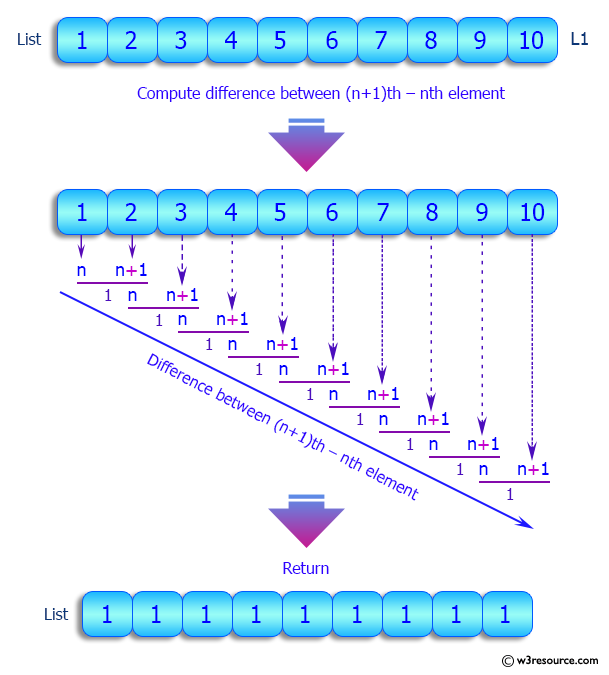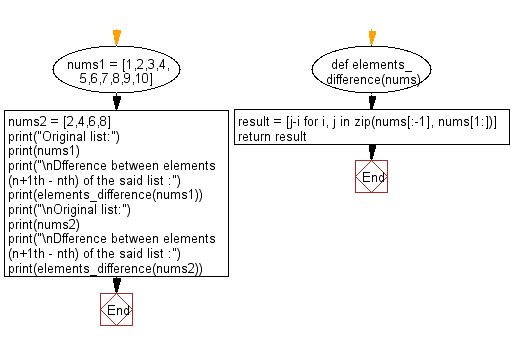﻿ Python: Find the difference between elements (n+1th – nth) of a given list of numeric values - w3resource

# Python: Find the difference between elements (n+1th – nth) of a given list of numeric values

## Python List: Exercise - 118 with Solution

Write a Python program to find the difference between elements (n+1th – nth) of a given list of numeric values.

Sample Solution:

Python Code:

``````def elements_difference(nums):
result = [j-i for i, j in zip(nums[:-1], nums[1:])]
return result

nums1 = [1,2,3,4,5,6,7,8,9,10]
nums2 = [2,4,6,8]

print("Original list:")
print(nums1)
print("\nDfference between elements (n+1th – nth) of the said list :")
print(elements_difference(nums1))
print("\nOriginal list:")
print(nums2)
print("\nDfference between elements (n+1th – nth) of the said list :")
print(elements_difference(nums2))

```
```

Sample Output:

```Original list:
[1, 2, 3, 4, 5, 6, 7, 8, 9, 10]

Dfference between elements (n+1th – nth) of the said list :
[1, 1, 1, 1, 1, 1, 1, 1, 1]

Original list:
[2, 4, 6, 8]

Dfference between elements (n+1th – nth) of the said list :
[2, 2, 2]
```

Pictorial Presentation:Flowchart:## Visualize Python code execution:

The following tool visualize what the computer is doing step-by-step as it executes the said program:

Python Code Editor:

Have another way to solve this solution? Contribute your code (and comments) through Disqus.

What is the difficulty level of this exercise?

Test your Programming skills with w3resource's quiz.

﻿

## Python: Tips of the Day

Returns True if the provided function returns True for every element in the list, False otherwise:

Example:

```def tips_every(lst, fn=lambda x: x):
return all(map(fn, lst))

print(tips_every([2, 4, 3], lambda x: x > 1))
print(tips_every([1, 2, 3]))
```

Output:

```True
True
```